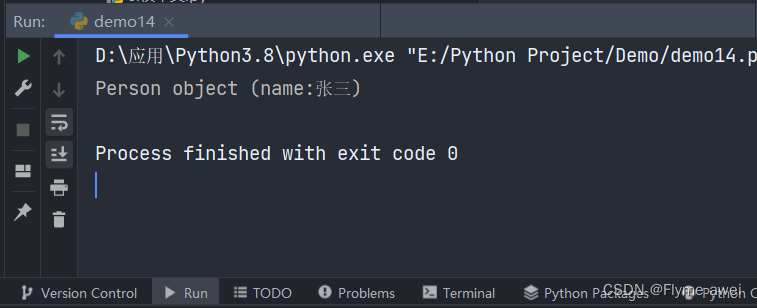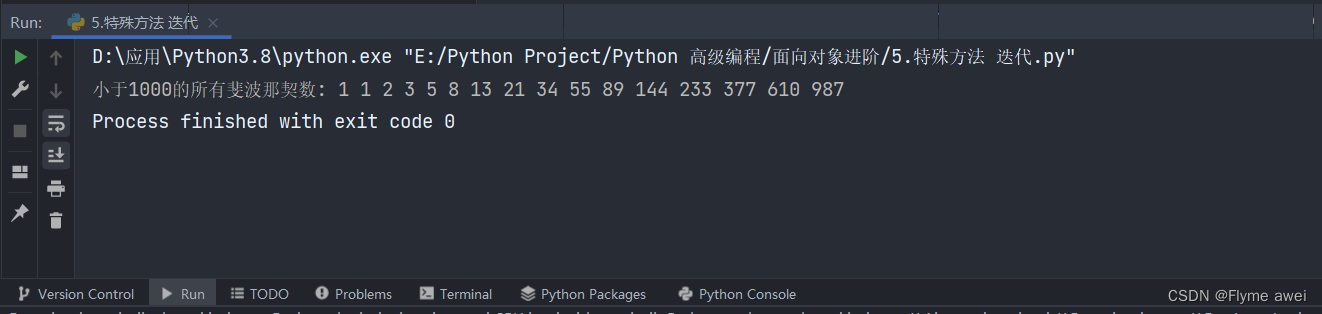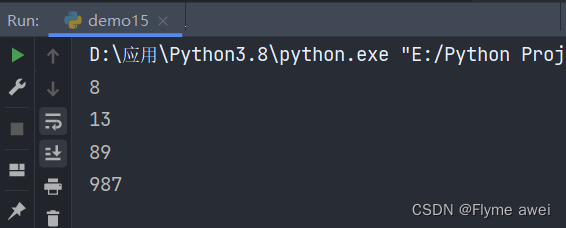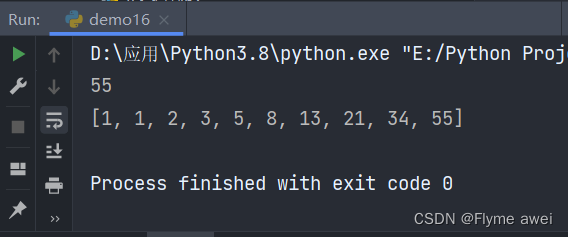> 文档中心 > 【Python面向对象进阶④】——定制类

# Python中的魔法方法@ author: Flyme awei

`__str__` 用于返回对象的描述
`__iter__` 使类可以迭代
`__getitem__` 按照下标获取类元素，例如list
`__getattr__` 调用类不存在的属性
`__call__` 类实例化默认调用方法

### 目录

• Python中的魔法方法
• 1.`__str__`
• 2.`__iter__`
• 3.`__getitem__`
• 4.`__getattr__`
• 5.`__call__`

`__slots__`我们已经知道怎么用了`，__len__`方法我们也知道是为了能让class作用于 `len()` 函数。

## 1.`__str__`

``>>> class Student(object):def __init__(self, name):self.name = name>>> print(Student('张三'))<__main__.Student object at 0x000001AC142D3370>>>> ``

``# -*- coding: utf-8 -*-# @author: Flyme awei # @Time  : 2022/7/1 21:55class Person(object):    def __init__(self, name): self.name = name    # 用于定制对象的描述信息    def __str__(self): return "Person object (name:%s)" % self.nameif __name__ == '__main__':    p = Person('张三')    print(p)``## 2.`__iter__`

``class Fib(object):    # Fib默认不是可迭代对象，变成一个可迭代对象，必须返回一个迭代器    def __init__(self): self.a, self.b = 0, 1  # 斐波那契数列前两个固定的值    # 重写 __iter__方法，Fib变为可迭代对象    def __iter__(self): return self    # 重写__next__方法，Fib就变成一个迭代器    def __next__(self): self.a, self.b = self.b, self.a + self.b  # 计算下一个值 if self.a > 1000:     raise StopIteration return self.aif __name__ == '__main__':    print('小于1000的所有斐波那契数:', end=' ')    for i in Fib(): print(i, end=' ')``## 3.`__getitem__`

`Fib`实例虽然能作用于for循环，看起来和list有点像，但是，把它当成`list`来使用还是不行，比如，取第5个元素：

``>>> Fib()Traceback (most recent call last): File "", line 1, in <module> TypeError: 'Fib' object does not support indexing>>>``

``# -*- coding: utf-8 -*-# @author: Flyme awei # @Time  : 2022/7/1 22:02class Fib(object):    # 重写__getitem__,Fib 可以类似于 list    def __getitem__(self, item): a, b = 1, 1 for x in range(item):     a, b = b, a+b return a``

``if __name__ == '__main__':    f = Fib()    print(f)    print(f)    print(f)    print(f) ````>>> list(range(100)[5:10])[5, 6, 7, 8, 9]``

``# -*- coding: utf-8 -*-# @author: Flyme awei # @Time  : 2022/7/2 17:36class Fib(object):    def __getitem__(self, item):  # # item是一个下标, 也有可能是一个切片 if isinstance(item, int):  # item 是一个 int 下标     a, b = 1, 1     for _ in range(item):   # rage(item) 用作循环次数  a, b = b, a+b     return a elif isinstance(item, slice):  # item 是一个切片（范围）     start = item.start     stop = item.stop     if start is None:  start = 0  # start初始值为 0      a, b = 1, 1     l = []     for _ in range(stop):  l.append(a)  a, b = b, a+b     return l``

``if __name__ == '__main__':    print(Fib())    print(Fib()[1:10])````>>> f[:10:2] [1, 1, 2, 3, 5, 8, 13, 21, 34, 55, 89]``

## 4.`__getattr__`

``class Student(object): def __init__(self): self.name = 'Michale'``

``>>> s = Student() >>> print(s.name) Michael >>> print(s.score) >Traceback (most recent call last): ... AttributeError: 'Student' object has no attribute 'score'``

``class Student(object):    def __init__(self): self.name = 'Michale'     def __getattr__(self, item): if item == 'score':     return 99     ``

``>>> s = Student() >>> s.name 'Michael' >>> s.score 99>>>``

``class Student(object):def __getattr__(self, start):if attr == 'age':return lambda : 25``

``>>> s,age()25``

``class Student(object):    def __getattr__(self, attr): if attr == 'age':     return lambda: 25 raise AttributeError('\'Student\' object has no attribute \'%s\'' % attr)``

## 5.`__call__`

``class Student(object):    def __init__(self, name): self.name = name     def __call__(self, *args, **kwargs): print('My name is %s.' % self.name)   ``

``>>> s = Student('awei')>>> s()  # self参数不要传入My name is awei.``

`__call__()` 还可以定义参数。对实例进行直接调用就好比对一个函数进行调用一样，所以你完全可以把对象看成函数，把函数看成对象，因为这两者之间本来就没啥根本的区别。

``>>> callable(Student()) True >>> callable(max) True >>> callable([1, 2, 3]) False >>> callable(None) False >>> callable('str') False``